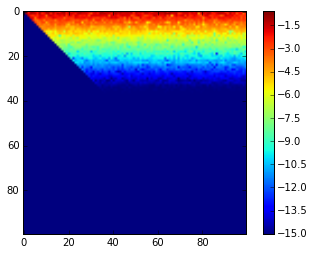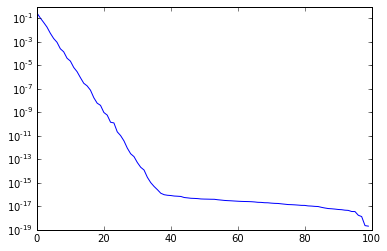# Rank-Revealing QR

Note: scipy.linalg, not numpy.linalg!

In :
import numpy as np
import scipy.linalg as la
import matplotlib.pyplot as pt


## Obtain a low-rank matrix

In :
n = 100
A0 = np.random.randn(n, n)
U0, sigma0, VT0 = la.svd(A0)
print(la.norm((U0*sigma0).dot(VT0) - A0))

sigma = np.exp(-np.arange(n))

A = (U0 * sigma).dot(VT0)

2.65180241653e-13



## Run the factorization

Compute the QR factorization with pivoting=True.

In :
Q, R, perm = la.qr(A, pivoting=True)


First of all, check that we've obtained a valid factorization

In :
la.norm(A[:, perm] - Q.dot(R), 2)

Out:
2.8038047842284552e-16

In :
la.norm(Q.dot(Q.T) - np.eye(n))

Out:
8.555792593659908e-15


Next, examine $R$:

In :
pt.imshow(np.log10(1e-15+np.abs(R)))
pt.colorbar()

Out:
<matplotlib.colorbar.Colorbar at 0x7f3a3fa000f0>Specifically, recall that the diagonal of $R$ in QR contains column norms:

In :
pt.semilogy(np.abs(np.diag(R)))

Out:
[<matplotlib.lines.Line2D at 0x7f3a3fa46780>]• In the case of scipy's transform, diagonal entries of $R$ are guaranteed non-increasing.
• But there is a whole science to how to choose the permutations (or other source vectors)
• and what promises one is able to make as a result of that